Engineering Mechanics - Planar Kinematics of a Rigid Body (PKRB)

Why Engineering Mechanics Planar Kinematics of a Rigid Body (PKRB)?

In this section you can learn and practice Engineering Mechanics Questions based on "Planar Kinematics of a Rigid Body (PKRB)" and improve your skills in order to face the interview, competitive examination and various entrance test (CAT, GATE, GRE, MAT, Bank Exam, Railway Exam etc.) with full confidence.

Where can I get Engineering Mechanics Planar Kinematics of a Rigid Body (PKRB) questions and answers with explanation?

IndiaBIX provides you lots of fully solved Engineering Mechanics (Planar Kinematics of a Rigid Body (PKRB)) questions and answers with Explanation. Solved examples with detailed answer description, explanation are given and it would be easy to understand. All students, freshers can download Engineering Mechanics Planar Kinematics of a Rigid Body (PKRB) quiz questions with answers as PDF files and eBooks.

Where can I get Engineering Mechanics Planar Kinematics of a Rigid Body (PKRB) Interview Questions and Answers (objective type, multiple choice)?

Here you can find objective type Engineering Mechanics Planar Kinematics of a Rigid Body (PKRB) questions and answers for interview and entrance examination. Multiple choice and true or false type questions are also provided.

How to solve Engineering Mechanics Planar Kinematics of a Rigid Body (PKRB) problems?

You can easily solve all kind of Engineering Mechanics questions based on Planar Kinematics of a Rigid Body (PKRB) by practicing the objective type exercises given below, also get shortcut methods to solve Engineering Mechanics Planar Kinematics of a Rigid Body (PKRB) problems.

Exercise :: Planar Kinematics of a Rigid Body (PKRB) - General Questions

• Planar Kinematics of a Rigid Body (PKRB) - General Questions
1.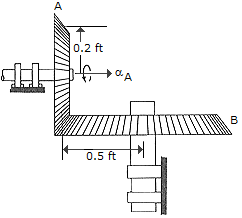Gear A is in mesh with gear B as shown. If A starts from rest and has a constant angular acceleration of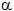A = 2 rad/s2, determine the tome needed for B to attain an angular velocity of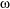B = 50 rad/s.

 A. t = 62.5 s B. t = 250.0 s C. t = 10.00 s D. t = 40.0 s

Explanation:

No answer description available for this question. Let us discuss.

2.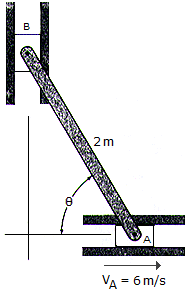The 2-m-long bar is confined to move in the horizontal and vertical slots A and B. If the velocity of the slider block at A is 6 m/s, determine the bar's angular velocity and the velocity of block B at the instant= 60°.

 A.AB = 3.46 rad/s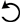, vB = 3.46 m/s 9 B.AB = 3.00 rad/s, vB = 3.00 m/s 9 C.AB = 3.00 rad/s, vB = 6.00 m/s 9 D.AB = 6.00 rad/s, vB = 10.39 m/s 9

Explanation:

No answer description available for this question. Let us discuss.

3.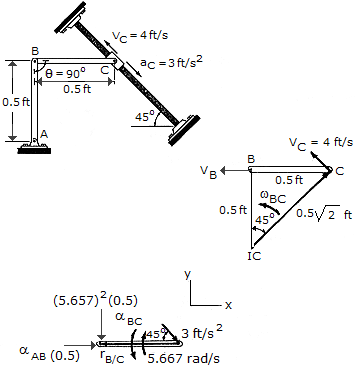Determine the angular acceleration of link BC at the instant= 90° if the collar C has an instantaneous velocity of vc = 4 ft/s and deceleration of ac = 3 ft/s2 as shown.

 A.BC = 36.2 rad/s2B.BC = 36.2 rad/s2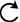C.BC = 27.8 rad/s2D.BC = 27.8 rad/s2Explanation:

No answer description available for this question. Let us discuss.

4.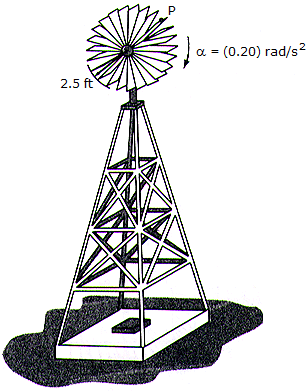During a gust of wind, the blades of the windmill are given an angular acceleration of= (0.2) rad/s2, whereis measured in radians. If initially the blades have an angular velocity of 5 rad/s, determine the speed of point P located at the tip of one of the blades just after the blade has turned two revolutions.

 A. vP = 5.60 ft/s B. vP = 19.87 ft/s C. vP = 14.05 ft/s D. vP = 18.81 ft/s

Explanation:

No answer description available for this question. Let us discuss.

5.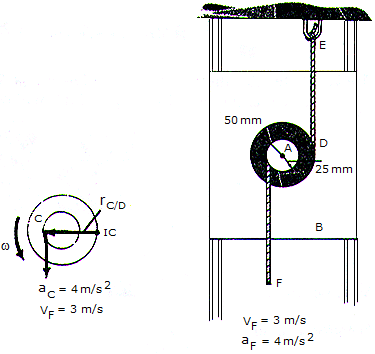The pulley os pin-connected to block B at A. As cord CF unwinds from the inner hub with the motion shown, cord DE unwinds from the outer rim. Determine the angular acceleration of the pulley at the instant shown.

 A.= 80.0 rad/s2 B.= 160.0 rad/s2 C.= 180.0 rad/s2 D.= 53.3 rad/s2